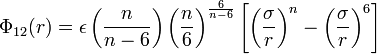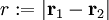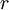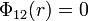# Difference between revisions of "N-6 Lennard-Jones potential"

The n-6 Lennard-Jones potential is a variant the more well known Lennard-Jones model (or from a different point of view, a particular case of the Mie potential). The potential is given by :$\Phi_{12}(r) = \epsilon \left( \frac{n}{n-6} \right)\left( \frac{n}{6} \right)^{\frac{6}{n-6}} \left[ \left(\frac{\sigma}{r} \right)^{n}- \left( \frac{\sigma}{r}\right)^6 \right]$

where

•$r := |\mathbf{r}_1 - \mathbf{r}_2|$
•$\Phi_{12}(r)$ is the intermolecular pair potential between two particles, "1" and "2".
•$\sigma$ is the diameter (length), i.e. the value of$r$ at which$\Phi_{12}(r)=0$
•$\epsilon$ is the well depth (energy)

## Melting point

An approximate method to locate the melting point is given in . See also .